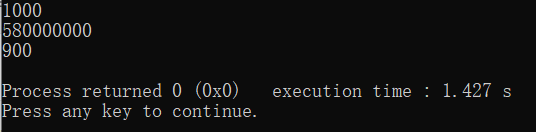# ACM/OI卡常技巧总结（clock大法好）

• NO.10: 特殊运算优化：

inline int inc(int x,int v,int mod){x+=v;return x>=mod?x-mod:x;}//代替取模+
inline int dec(int x,int v,int mod){x-=v;return x<0?x+mod:x;}//代替取模-



inline int Abs(int a){//绝对值优化
{
int b=a>>31;
return (a+b)^b;
}


• NO.9：前置++/--运算符：(有利无弊)

• NO.8: if()else语句比()?():()语句慢(但慢的不多，在判断较少的时候还是用if吧)。

• NO.7: 内联：

inline add(int u,int v)
{
star[++cnt].to=v;
star[cnt].nxt=head[u];
head[u]=cnt;
}


struct haha{
int v,x;
inline bool operator < (haha tar){//强制内联
return v<tar.v;
}
}lala[MAXN+1];

• NO.6： 使用局部变量的效率比使用静态变量要高。

• NO.5：优化STL

#include<bits/stdc++.h>
using namespace std;
#define reg register
static char space,*sp=space;
template<typename T>
struct myalloc:allocator<T>{
myalloc(){}
template<typename T2>
myalloc(const myalloc<T2> &a){}
template<typename T2>
myalloc<T>& operator=(const myalloc<T2> &a){return *this;}
template<typename T2>
struct rebind{typedef myalloc<T2> other;};
inline T* allocate(size_t n){
T *result=(T*)sp;sp+=n*sizeof(T);
return result;
}
inline void deallocate(T* p,size_t n){}
};

list<int,myalloc<int> > L;vector<double,myalloc<double> > vec //容量的定义


• NO.4: I/O优化

scanfcin快得多，printfcout快得多，如果你不知道就……就现在知道了

void read(int &x)
{
int f=1;x=0;char s=getchar();
while(s<'0'||s>'9'){if(s=='-')f=-1;s=getchar();}
while(s>='0'&&s<='9'){x=x*10+s-'0';s=getchar();}
x*=f;
}


inline char get_char(){//超级快读
static char buf,*p1=buf,*p2=buf;
return p1==p2&&(p2=(p1=buf)+fread(buf,1,1000000,stdin),p1==p2)?EOF:*p1++;
}
inline int read(){
int num=0;
char c;
while(isspace(c=get_char()));
while(num=num*10+c-48,isdigit(c=get_char()));
return num;
}

• NO.3: register

• NO.2: #pragma GCC optimize(2)（请勿在NOIP中作死）

• NO.1: clock()大法好！

clock()函数可以返回当前的运行时间，也就是说在进行循环或者爆搜的时候超时，时间限制为 1 s 1s 我们就可以判断一下当前是否超时，如果快要超时了就立刻break出来，避免TLE

#include<iostream>
#include<cstdio>
#include<algorithm>
#include<ctime>

using namespace std;

int start = clock();

int main(){
cout<<CLOCKS_PER_SEC<<endl;

for(int i = 0; ; ++i){
if(i % 100000 == 0 && clock() - start >= CLOCKS_PER_SEC * 0.9){
cout<<i<<endl;
cout<<clock() - start <<endl;
exit(0);
}
}
return 0;
}• clock()函数本身运行比较慢，所以要尽量少用，少判断，所以我在代码的判断if一行加上了i % 100000000 == 0
• clock()在不同的系统表示的时间单位不同，在liuns上是1000000为1s，而windows中经过测试为1000为1s

04-241万+01-108742
10-25216
09-16391
08-013153
08-0255
10-1583
05-141万+
11-073326
11-131279
12-221186
03-2576
12-111220
08-03499
08-09339
05-292603
01-31162
10-058209
04-15146
©️2020 CSDN 皮肤主题: 撸撸猫 设计师:C马雯娟